### What are transcendental numbers?

Transcendental numbers are defined in contrast to algebraic numbers: we say that a number is algebraic if it is the solution of a polynomial equation all whose coefficients are integers, that is to say, an equation consisting of a sum of powers of the unknown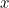which can be multiplied by integers. Examples are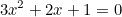or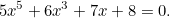Thus, for example, the number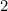is algebraic because it is the solution of the equation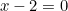. In a similar way, any whole number or any fraction (a rational number) is also algebraic: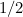, for instance, is the solution of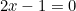. Another example of an algebraic number is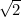: this is a solution of the equation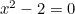.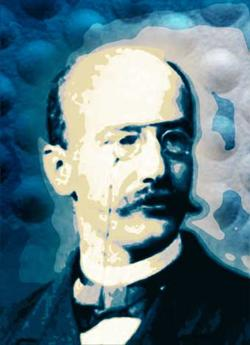Ferdinand von Lindemann.

Any number that is not algebraic is called transcendental. Since every rational number is algebraic, it follows that every transcendental number is necessarily irrational (that is, not rational). But not every irrational number is transcendental: takefor example (see Maths in a minute: The square root of 2 is irrational).

Probably the most famous transcendental number is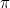(another is; see Where does e come from and what does it do?). Even beforewas proven to be transcendental, mathematicians had long had the creeping suspicion that it was somehow unlike most of the other numbers that they encountered. It was certainly known thatis irrational, but its weirdness as a number seemed to go beyond this.

Despite the fact that the notions of algebraic and transcendental numbers had first been defined by Leonhard Euler in the 18th century, it was not until 1882 that the German mathematician Ferdinand von Lindemann demonstrated thatis in fact transcendental. As well as proving once and for all that it is not possible to square the circle (see Mathematical mysteries: Transcendental meditation), this also explained whyseemed so unlike other numbers: because we can't write down equations of which they are solutions, transcendental numbers are harder to "get hold of" than algebraic ones. In essence, an equation for a number provides us with a finite process by which we can construct that number; in the case of transcendental numbers, we have no such process.

### Hilbert's seventh problem

At the end of the nineteenth century,was one of only very few examples of transcendental numbers that were known. And yet mathematicians knew that there are in fact very many more transcendental numbers than algebraic: the transcendental numbers are uncountably infinite, whereas the algebraic numbers are countable (see Maths in a minute: Counting numbers). More examples of transcendental numbers were needed. Indeed, what would be more useful would be a new method for constructing examples of transcendental numbers. The respected German mathematician David Hilbert took account of this need when, at the International Congress of Mathematicians (ICM) in 1900, he presented a now famous list of problems that he thought ought to be tackled by mathematicians in the coming decades. Finding a method for constructing transcendental numbers was the seventh problem on this list.

Hilbert had a conjecture for how this seventh problem might be solved. Suppose that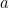is an algebraic number that is neither 0 nor 1 (to avoid trivial cases), and suppose thatis an irrational algebraic number. Following on from a similar conjecture made by Euler (wherewas assumed merely to be rational), Hilbert conjectured that in this situation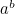is necessarily transcendental. His seventh problem was to find a rigorous proof of this. If it could be proved to be true, then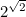, for example, would be transcendental, despite the fact that neithernoris itself transcendental. Mathematicians would thus at last have a means of constructing further transcendental numbers from better-known building blocks (that is, algebraic numbers).

The person to finally provide a rigorous proof of the seventh problem was the Russian mathematician A. O. Gel'fond. Spurred on by the proof in 1930 by his fellow Russian Rodion Kuzmin thatis indeed transcendental, Gel'fond had considered the more general case. He published a full solution in 1934.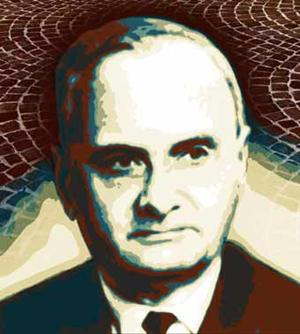Aleksandr Gel'fond.

### Why politics?

A proof of such an important conjecture would normally afford its author centre stage in international mathematics, but Gel'fond became a victim of political tensions. He had been invited to present his proof at the International Congress of Mathematicians in Oslo in 1936. That particular congress, however, proved a melting pot of different agendas. While mathematicians from Nazi-led Germany turned out in force to showcase the best of "Aryan mathematics", the Soviet delegation was conspicuous by its absence. In an atmosphere in which scientists' foreign connections were increasingly regarded with suspicion by the Soviet authorities, each of the delegates expected from the USSR, including Gel'fond, had been denied permission to travel. The world's mathematicians had to be content merely to read Gel'fond's solution of Hilbert's problem (it had been published in French), rather than see it presented at a blackboard by the author. (You can find out more about international maths and politics in this article.)

Gel'fond was finally able to attend an ICM in 1966, when it was held in his home town of Moscow, although he does not appear to have delivered a lecture. As far as we know, this is the only ICM that he ever attended. The congress was certainly attended by a small number of mathematicians who had been in Oslo thirty years earlier, but we can only speculate about the interactions that resulted when they were finally able to meet Gel'fond in Moscow. It is interesting to note that the solution of a generalisation of Hilbert's seventh problem, sometimes termed the Gel'fond conjecture, earned a Fields Medal, one of the most prestigious prizes in mathematics, for the English mathematician Alan Baker at the 1970 ICM in Nice.